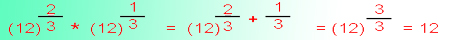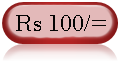Web Development : Starting from Concept, HTML, CSS, Web Site Develpment to Website Launching.
Written by: Sanjay Srivastava
B.Tech (Honours);IIT Kharagpur
PMP ; PMI USA

Class - VIII - Maths:- INDEX

1. Squares & Square Roots
2. Cubes & Cube Roots
4. Profit Loss & Discount
5. Compound Interest
6. Algebraic Identities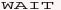7. Polynomials8. Linear Equations9. Parallel Lines10. Types of Quadrilaterals11. Construction of Quadrilaterals12. Circles13. Areas14. Surface Areas15. Volumes16. StatisticsClass - VIII - Maths

## Solve Exponential Equations (Page 2/4)

Question - Type 2 - Find the value of this exponent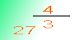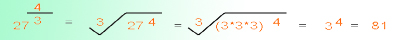Question - Type 3 - Find the value of this exponent equation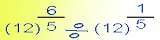Answer - 3- Refer Important tip no (3) regarding exponent equation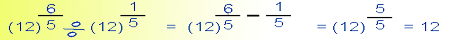Question - Type 4 - Find the value of this exponent equation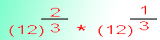Answer - 4- Refer Important tip no (2) regarding exponent equation